# Find the inverse of the element x^2 + x^3 + 1 in the field F2[x] / (x^4 + x^3 +1)

Find the inverse of the element x^2 + x^3 + 1 in the field F2[x] / (x^4 + x^3 +1)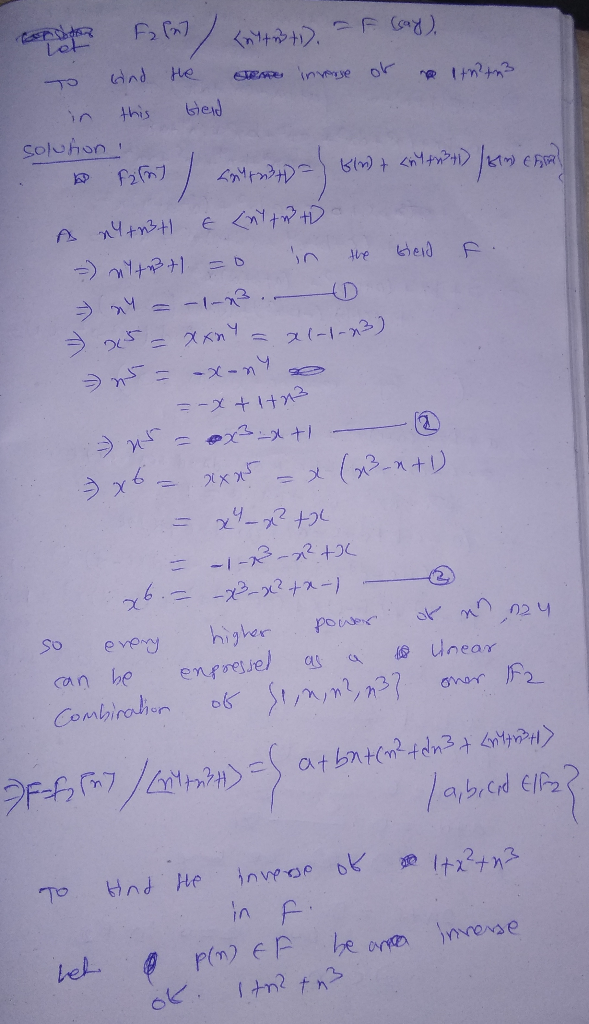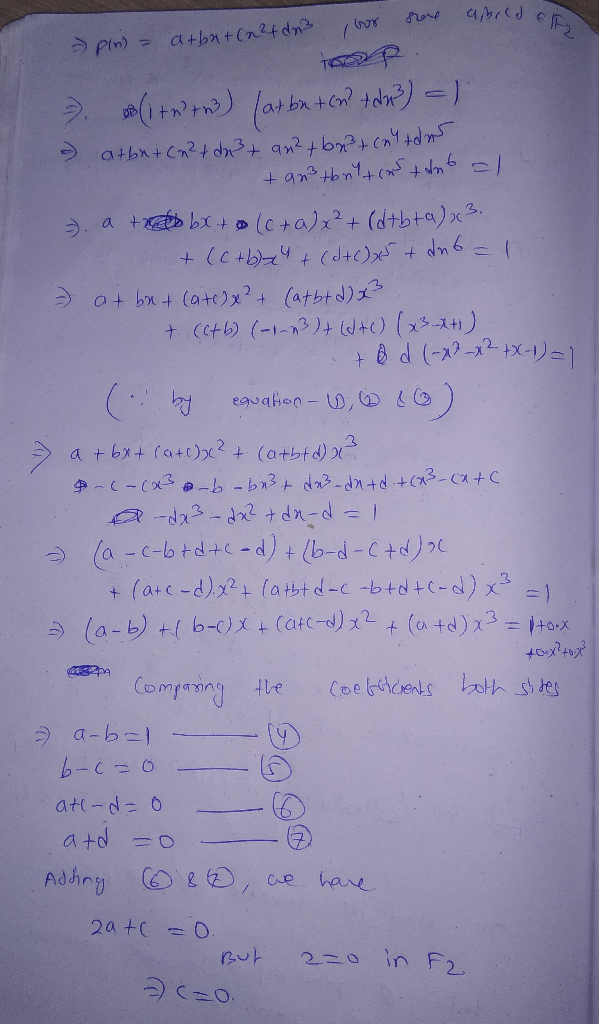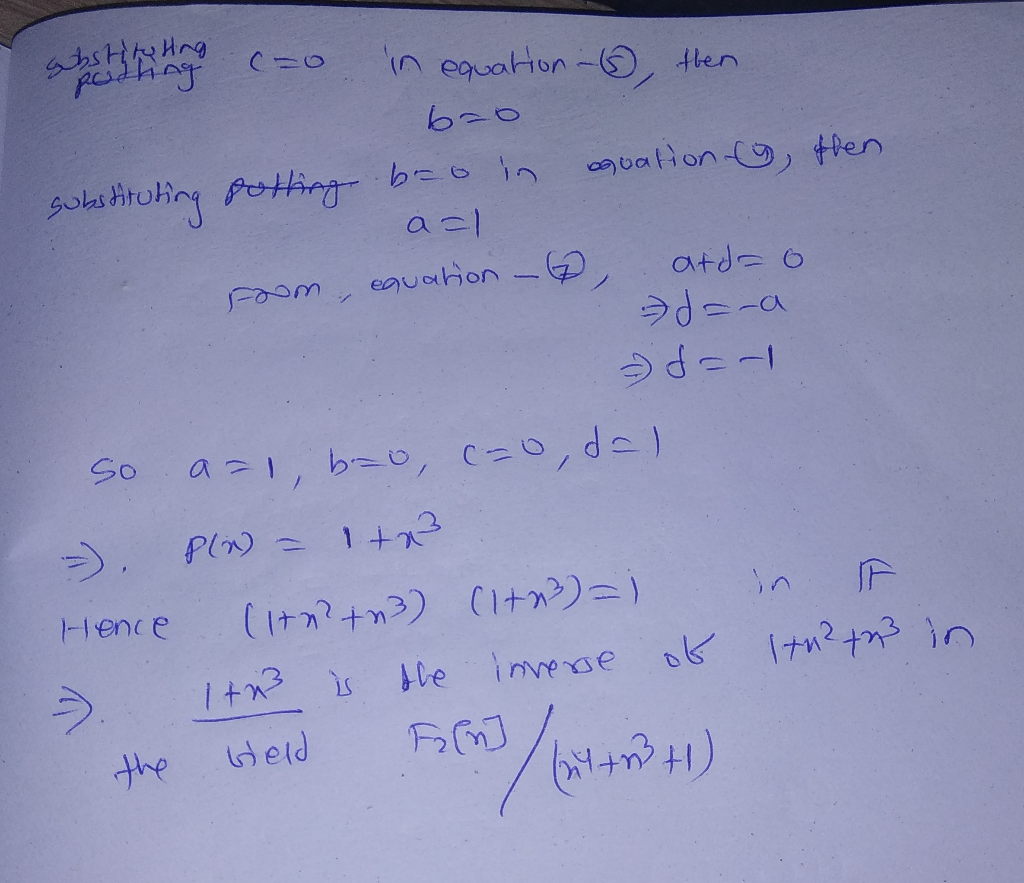##### Add Answer of: Find the inverse of the element x^2 + x^3 + 1 in the field F2[x] / (x^4 + x^3 +1)
Similar Homework Help Questions
• ### 5. Create a 4 x 4 matrix , called A, of randonm integers between 1 and 10. 1. find the transpose of A 2. find the trace of A 3. find the inverse of A 5. Create a 4 x 4 matrix , called A, of...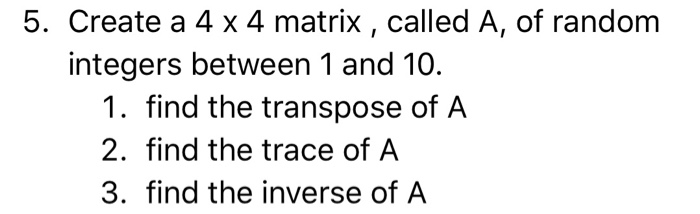5. Create a 4 x 4 matrix , called A, of randonm integers between 1 and 10. 1. find the transpose of A 2. find the trace of A 3. find the inverse of A 5. Create a 4 x 4 matrix , called A, of randonm integers between 1 and 10. 1. find the transpose of A 2. find the trace of A 3. find the inverse of A

• ### Find the inverse of the function f(x)= X^1/3+2

Find the inverse of the function f(x)= X^1/3+2.

• ### Find the inverse of the function f(x)= x^1/3 +2

Find the inverse of the function f(x)= x^1/3 +2.

• ### find the inverse of the function f(x)=x^1/3+2

find the inverse of the function f(x)=x^1/3+2

• ### Find the inverse of f(x)= (x-3)/(x+2) and f(x)= (8x-4)/(2x+6)

Find the inverse of f(x)= (x-3)/(x+2) and f(x)= (8x-4)/(2x+6).

• ### Find the inverse of the matrix( 1 -2 3 ) 2 -3 8 4 -7 11

Find the inverse of the matrix( 1 -2 3 ) 2 -3 8 4 -7 11

• ### Find the inverse of f(x)=x^3 + 2

Find the inverse of f(x)=x^3 + 2

• ### find the inverse z transform X(z) = 1-2-3 with <1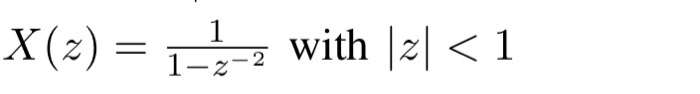find the inverse z transform X(z) = 1-2-3 with <1

• ### find the inverse of y = (2^x) -3

find the inverse of y = (2^x) -3

• ### 2) (12) f:R-(3/2)-R-10, (x) 1/(3 2x) g:R--21->R-1o), g (x)1/ (x 2) h:R-(-4/3]-R-(1/3), h(x) (f o g) (x) Verify if h(x) is one to one and onto. If it is, find the inverse function of h(x)....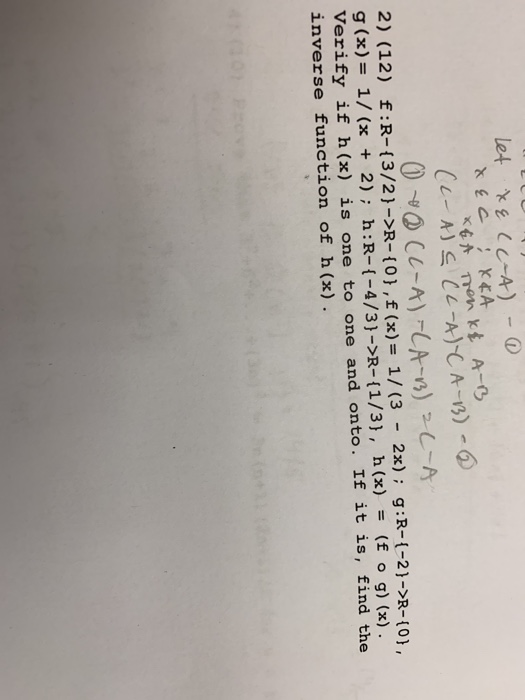2) (12) f:R-(3/2)-R-10, (x) 1/(3 2x) g:R--21->R-1o), g (x)1/ (x 2) h:R-(-4/3]-R-(1/3), h(x) (f o g) (x) Verify if h(x) is one to one and onto. If it is, find the inverse function of h(x). 2) (12) f:R-(3/2)-R-10, (x) 1/(3 2x) g:R--21->R-1o), g (x)1/ (x 2) h:R-(-4/3]-R-(1/3), h(x) (f o g) (x) Verify if h(x) is one to one and onto. If it is, find the inverse function of h(x).

Need Online Homework Help?